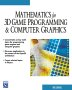# Maths - Trigonometry - Infinite series

## Calculating Trig functions

We can calculate trig functions from an infinite series as follows:

sin(a) = a - ( a 3 / 3!) + ( a 5 / 5!) - ( a 7 / 7!) + ...
cos(a) = 1 - ( a 2 / 2!) + ( a 4 / 4!) - ( a 6 / 6!) + ...
tan(a) = a + ( a 3 / 3) + ( 2a5 / 15) + ...

inverse functions

sin-1(x) = x + ( x3 / 3!) + ( 9x5 / 5!) + (225 x7 / 7!) + ...
cos-1(x) = PI/2 - x - ( x3 / 3!) - (9 x5 / 5!) - ( 225 x7 / 7!) + ...
tan-1(x) = x - ( x3 / 3) + ( x5 / 5) - ( x7 / 7) + ...

where:

• a = angle in radians
• n! = 1*2*3* ... *n = n factorial = product of all integers upto n
• sin-1(x) = arcsin(x) = inverse of sin function (similar for cos & tan)
• PI = 3.14159

This provides a method to allow maths libraries to calculate trig functions to the level of accuracy required.

 metadata block see also: Correspondence about this page Book Shop - Further reading. Where I can, I have put links to Amazon for books that are relevant to the subject, click on the appropriate country flag to get more details of the book or to buy it from them.Mathematics for 3D game Programming - Includes introduction to Vectors, Matrices, Transforms and Trigonometry. (But no euler angles or quaternions). Also includes ray tracing and some linear & rotational physics also collision detection (but not collision response). Other Math Books

This site may have errors. Don't use for critical systems.